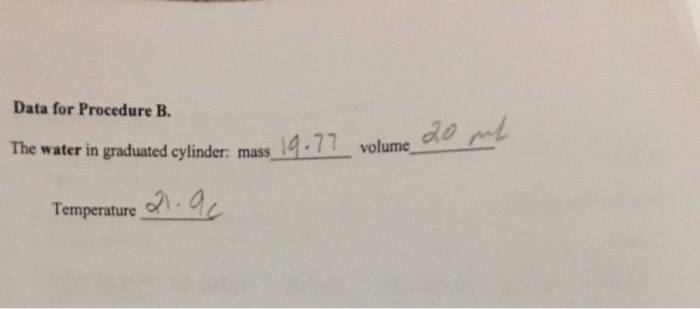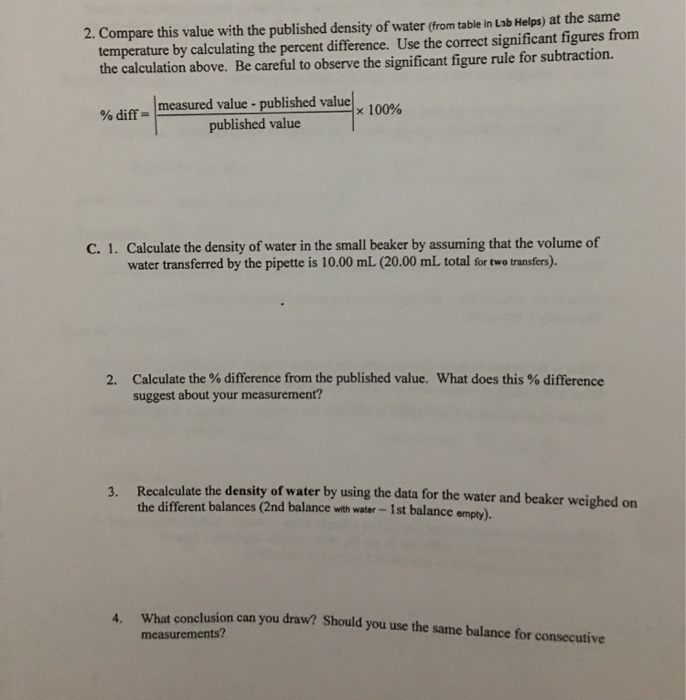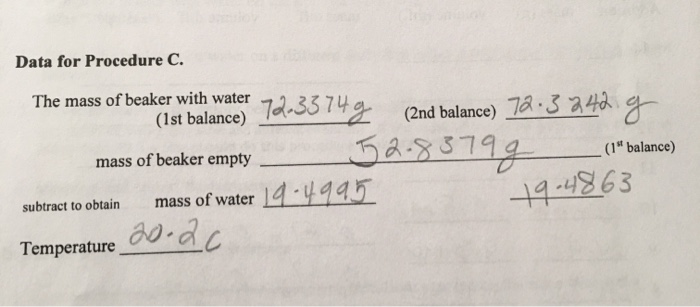### Create an Account

Home / Questions / Data for Procedure B. The water in graduated cylinder: mass Temperature 21.9c 2. Compare t...

# Data for Procedure B. The water in graduated cylinder: mass Temperature 21.9c 2. Compare this value with the published density of water (from table in Lab Helps) at the same temperature by calculatingpart C 1-4Show transcribed image textData for Procedure B. The water in graduated cylinder: mass Temperature 21.9c
2. Compare this value with the published density of water (from table in Lab Helps) at the same temperature by calculating the percent difference. Use the correct significant figures from the calculation above. Be careful to observe the significant figure rule for subtraction. % diff measured value - published value published value C. 1. Calculate the density of water in the small beaker by assuming that the volume of water transferred by the pipette is 10.00 mL (20.00 mL total for two transfers). 2. Calculate the % difference from the published value. What does this % difference suggest about your measurement? 3. Recalculate the density of water by using the data for the water and beaker weighed on the different balances (2nd balance with water - ist balance empty). What conclusion can you draw? Should you use the same balance for consecutive measurements?
Data for Procedure C. The mass of beaker with water, (1st balance) ST 72-3374g (2nd balance) 72.3240 g mass of beaker empty 52.83799 (1" balance) subtract to obtain mass of water 19-4995 Temperature 19.4863part C 1-4Show transcribed image textData for Procedure B. The water in graduated cylinder: mass Temperature 21.9c
2. Compare this value with the published density of water (from table in Lab Helps) at the same temperature by calculating the percent difference. Use the correct significant figures from the calculation above. Be careful to observe the significant figure rule for subtraction. % diff measured value - published value published value C. 1. Calculate the density of water in the small beaker by assuming that the volume of water transferred by the pipette is 10.00 mL (20.00 mL total for two transfers). 2. Calculate the % difference from the published value. What does this % difference suggest about your measurement? 3. Recalculate the density of water by using the data for the water and beaker weighed on the different balances (2nd balance with water - ist balance empty). What conclusion can you draw? Should you use the same balance for consecutive measurements?
Data for Procedure C. The mass of beaker with water, (1st balance) ST 72-3374g (2nd balance) 72.3240 g mass of beaker empty 52.83799 (1" balance) subtract to obtain mass of water 19-4995 Temperature 19.4863

Jun 17 2021 View more View LessSubscribe To Get Solution